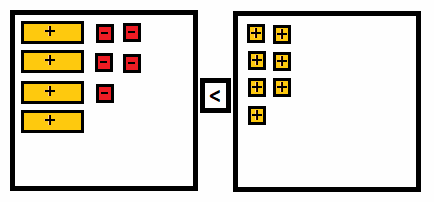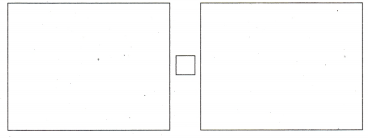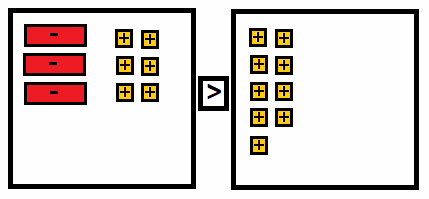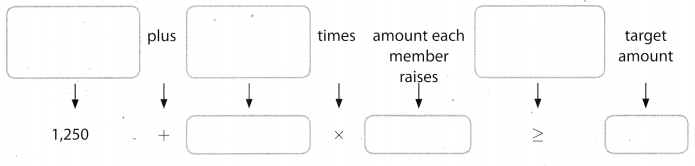# Texas Go Math Grade 7 Lesson 8.3 Answer Key Writing Two-Step Inequalities

Refer to our Texas Go Math Grade 7 Answer Key Pdf to score good marks in the exams. Test yourself by practicing the problems from Texas Go Math Grade 7 Lesson 8.3 Answer Key Writing Two-Step Inequalities.

## Texas Go Math Grade 7 Lesson 8.3 Answer Key Writing Two-Step Inequalities

Modeling Two-Step inequalities
You can use algebra tiles to model two-step inequalities.

Use algebra tiles to model 2k + 5 ≥ -3.

A. Using the line on the mat, draw in the inequality symbol shown in the inequality.B. How can you model the left side of the inequality?

C. How can you model the right side of the inequality?

D. Use algebra tiles or draw them to model the inequality on the mat.

Reflect

Question 1.
Multiple Representations How does your model differ from the one you would draw to model the equation 2k + 5 = -3?
On each side we put the same number of tiles as in the model of equation 2k + 5 = -3.
Only difference is that instead of equality sign, we have inequality sign.

Question 2.
Why might you need to change the inequality sign when you solve an inequality using algebra tiles?
The reason when you need to change the inequality sign when you solve an inequality using algebra tiles is when the variable is negative. When solving an inequality, wherein the variable is with a negative coefficient, the inequality sign needs to be changed. When an inequality have a negative variable.

Question 3.
The 45 members of the glee club are trying to raise at least $6,000 so they can compete in the state championship. They already have$1240 What inequality can you write to find the amount each member must raise, on average, to meet the goal?
Let x be the amount each member must raise.
$1240 + 45 ∙ x ≥$6000

Question 4.
Ella has $40 to spend at the State Fair. Admission is$6 and each ride costs $3. Write an inequality to find the greatest number of rides she can go on. Answer: Let æ be the number of rides Ella can go on.$6 + $3 ∙ x ≤$40

Write a real-world problem for each inequality.

Question 5.
3x + 10 > 30
In order to get a bonus this month, leon must sell more than 30 newspaper subscriptions
He sold 10 subscriptions in the first week of the month.
How many subscriptions must leon sell per week if there is three more weeks of the month?

Question 6.
5x – 50 ≤ 100
You have to pay $50 rent a spot at the flea market to sell your stuff. You make$5 profit each one you sell.
How many do you have to sell to make $100 profit or less. Texas Go Math Grade 7 Lesson 8.3 Guided Practice Answer Key Draw algebra tiles to model each two-step inequality. (Explore Activity) Question 1. 4x – 5 < 7Answer:On the left side of the mat we put 4 positive variable and 5 – 1 tiles. On the right side we put 7 + 1 tiles, and between is the sign of inequality. Question 2. -3x + 6 > 9Answer:On the left side of the mat we put 3 negative variable and 6 + 1 times. On the right side we put 7 +1 tiles, and between is the sign of inequality. Question 3. The booster club needs to raise at least$7,000 for new football uniforms. So far, they have raised $1,250. Write an inequality to find the average amounts each of the 92 members can raise to meet the club’s objective. (Example 1) Let a represent the amount each member must raise.The inequality that represents the situation is _____ Answer:Amount to be raised:$7000
Amount already raised: $1250 Number of members: 92 1250 + 12 ∙ a ≥ 7000 Question 4. Analyze what each part of 7x – 18 ≤ 32 means mathematically. (Example 2) x is ____________________. 7x is ________ 18 means that ________________________ ≤ 32 means that ________________________ Answer: -Solution. -Solution multiplied by 7. -Subtract from 7x. -At most 32. Question 5. Write a real-world problem to represent 7x – 18 ≤ 32. Answer: Lenna has$32 and wants to buy the same Christmas sweaters for 7 friends.
Also she has a gift card of $18 that she plans to use. How much at most can it cost one sweater? Essential Question Check-In Question 6. Describe the steps you would follow to write a two-step inequality you can use to solve a real-world problem. Answer: The steps in writing a two-step inequality to solve a real-world problem: • 1. Read the problem carefully and identify what is needed. Assign a variable for the said needed information. • 2. Write down the important information that can be used to help to write an inequality. • 3. Use words in the problem to tie the information together. Translate these words into numbers and variables in order to make an inequality. The steps in writing a two-step inequality to solve a real-world problem. Texas Go Math Grade 7 Lesson 8.3 Independent Practice Answer Key Question 7. Three friends earned more than$200 washing cars. they paid their parents $28 for supplies and divided the rest of money equally. Write an inequality to find possible amounts each friend earned. Identify what your variable represents. Answer: Three friends paid their parents$28 for supplies. and they earned more than $200 washing cars. Let x be possible amount each friend earned. 3x –$28 > $200 Question 8. Nick has$7.00. Bagels cost $0.75 each, and a small container of cream cheese costs$1.29. Write an inequality to find the numbers of bagels Nick can buy. Identify what your variable represents.
Container of cream cheese costs$1.29. and each bagels cost$0.75. Let x be the number of bagels Nick can buy with his $7.$075 ∙ x + $1.29 <$7

Question 9.
Chet needs to buy 4 work shirts, all costing the same amount. The total cost before Chet applies a $25 gift certificate can be no more than$75. Write an inequality to find the possible amounts that Chet pays per shirt. Identify what your variable represents.
Assign a variable for the unknown value. Let x be the cost of each shirt. The inequality for the given problem is:
4x + 25 ≤ 75

Question 10.
Due to fire laws, no more than 720 people may attend a performance at Metro Auditorium. The balcony holds 120 people. There are 32 rows on the ground floor, each with the same number of seats. Write an inequality to find the numbers of people that can sit in a ground-floor row if the balcony is full. Identify what your variable represents.
We have to sum the number of people on the balcony, and how many people can sit in 32 rows on the ground floor, and that sum can not be greater than 720. Let x be the number of people in the rows on the ground floor.
32 ∙ x + 120 < 720

Question 11.
Liz earns a salary of $2,100 per month, plus a commission of 5% of her sales. She wants to earn at least$2,400 this month. Write an inequality to find amounts of sales that will meet her goal. Identify what your variable represents.
We have to sum Liz’s month salary and 5% commission of hers sales, to find the amount. of sales that will meet her goal. Let x be the amount of sales.
$2100 + 5% ∙ x <$2400 5% we can write as a fraction 1/20.
$2100 + $$\frac{1}{20}$$ ∙ x <$2400
$2100 + $$\frac{x}{20}$$ <$2400

Question 12.
Lincoln Middle School plans to collect more than 2,000 cans of food in a food drive. So far, 668 cans have been collected. Write an inequality to find numbers of cans the school can collect on each of the final 7 days of the drive to meet this goal. Identify what your variable represents.
We have to sum 668 cans that had been collected, and how many cans should be collected in 7 days, that the school achieve the plan. Let x be the number of cans the school should collect each day.
668 + 7 ∙ x > 2000

Question 13.
Joanna joins a CD club. She pays $7 per month plus$10 for each CD that she orders. Write an inequality to find how many CDs she can purchase in a month if she spends no more than $100. Identify what your variable represents. Answer: We have to sum$7 and the number od CDs. each costs $10, that Joanna can purchase in a month with no more than$100. Let x be the number of CDs.
$7 +$10 ∙ x < $100 Question 14. Lionel wants to buy a belt that costs$22. He also wants to buy some shirts that are on sale for $17 each. He has$80. What inequality can you write to find the number of shirts he can buy? Identify what your variable represents.
We have to sum the price of the belt $22 and how many shirts, that costs$17, Lionel can buy, and that sum can be at most $80. Let x be the number of shirts Lionel can buy.$22 + $17 ∙ x ≤$80

Question 15.
Write and solve a real-world problem that can be represented by 15x – 20 ≤ 130.
Given inequality in problem: 15x – 20 ≤ 130
15x – 20 ≤ 130 (Given)
15x – 20 + 20≤ 130 + 20 (Adding 20 on both side)
15x ≤ 150 (Simplifying)
x ≤ $$\frac{150}{15}$$ (Dividing both side by 15)
x ≤ 10 (SoLution)
x ∈ (-∞, 10]Analyze Relationships Write >, <, ≥, or ≤ in the blank to express the given relationship.

Question 16.
m is at least 25 m __________ 25
m 25

Question 17.
k is no greater than 9 k __________ 9
k 9

Question 18.
p is less than 48 p __________ 48
p < 48

Question 19.
b is no more than -5 b __________ -5
p -5

Question 20.
h is at most 56 h __________ 56
h 56

Question 21.
w is no less than 0 w __________ 0
w 0

Question 22.
Critical Thinking Marie scored 95, 86, and 89 on three science tests. She wants her average score for 6 tests to be at least 90. What inequality can you write to find the average scores that she can get on her next three tests to meet this goal? Use s to represent the lowest average score.
Let s represents the lowest average score on next three tests Marie have to take.
$$\frac{95+86+89+s}{6}$$ ≥ 90

Question 23.
Communicate Mathematical Ideas Write an inequality that expresses the reason the lengths 5 feet, 10 feet, and 20 feet could not be used to make a triangle. Explain how the inequality demonstrates that fact.
The triangle inequality states that the sum of the lengths of any two sides of a triangle must be greater than or equal to the length of the third side. Let a, b and c be the sides of the triangle. The inequalities that should be satisfied are:
a + b ≥ c
a + c ≥ b
b + c ≥ a
Although in our case we have
10 + 20 > 5
20 + 5 > 10
the sum of the lengths of the two shorter sides is less than the length of the third side
5 – 10 < 20
Hence, these lengths could not be used to make a triangle.

Question 24.
Analyze Relationships The number m satisfies the relationship m < 0. Write an inequality expressing the relationship between -m and 0. Explain your reasoning.
The number m is less than 0, so m could be 1, -2, -3 etc. If we take negative value of the number m, we will have
-(-1) = 1, -(- 2) = 2, -(- 3) = 3 etc. Hence, -m will be greater than 0.
Also, we could just multiply inequality m < 0 by (- 1). Because we are multiplying by a negative number, we have to reverse direction of the inequality.
m < 0
Multiply by -1 both sides.
-m > 0

Question 25.
Analyze Relationships The number n satisfies the relationship n > 0. Write three inequalities to express the relationship between n and $$\frac{1}{n}$$.
If 0 < n < 1, than the relationship between n and $$\frac{1}{n}$$ is
n < $$\frac{1}{n}$$
If n ≤ 1, than the relationship between n and $$\frac{1}{n}$$ is
n ≤ $$\frac{1}{n}$$
If n > 1, than the relationship between n and $$\frac{1}{n}$$ is
n > $$\frac{1}{n}$$